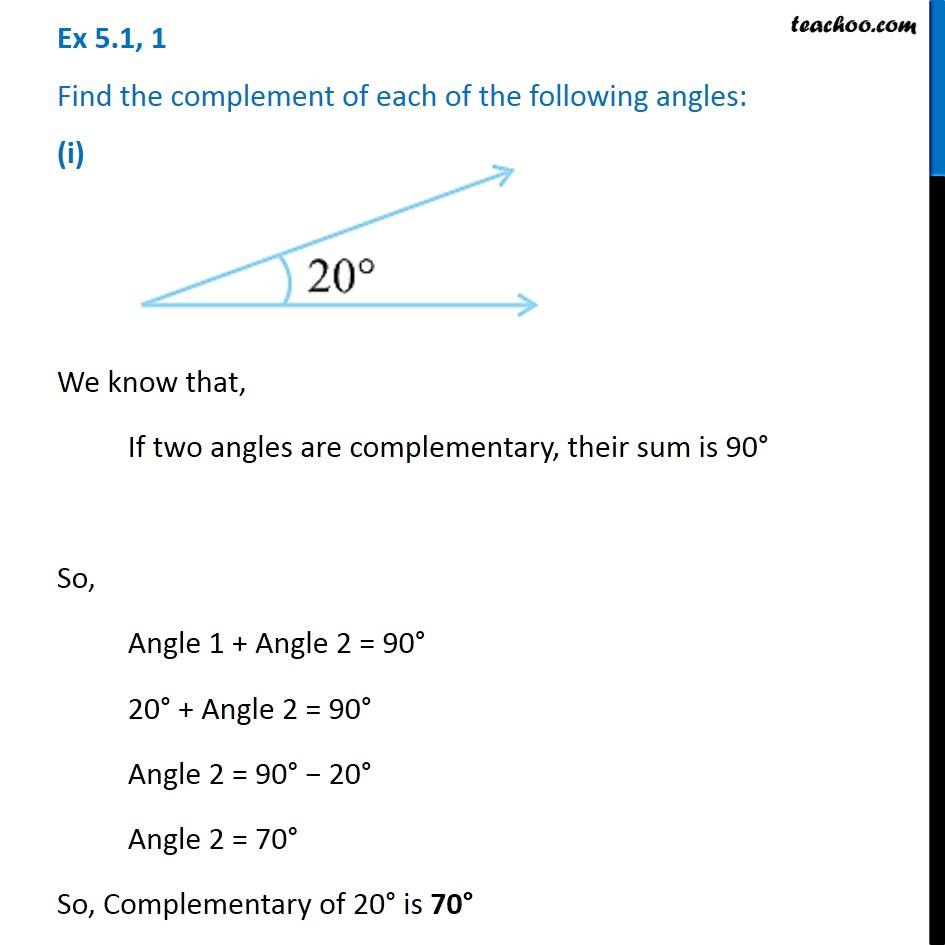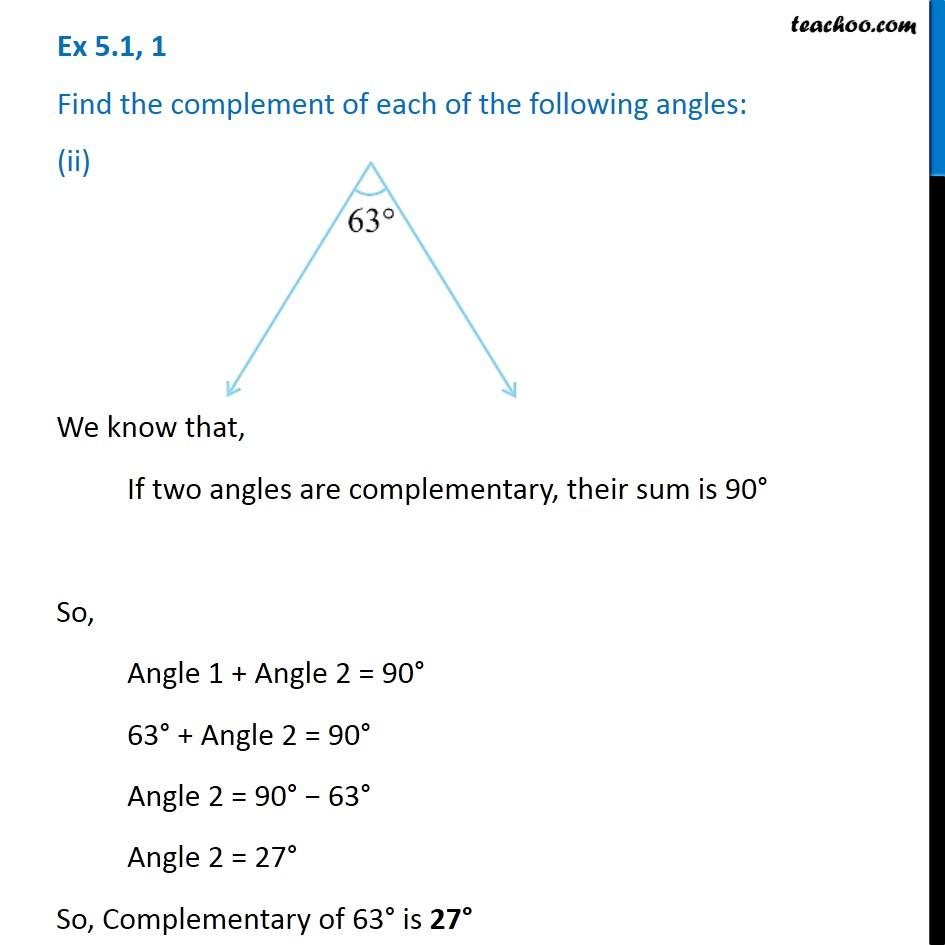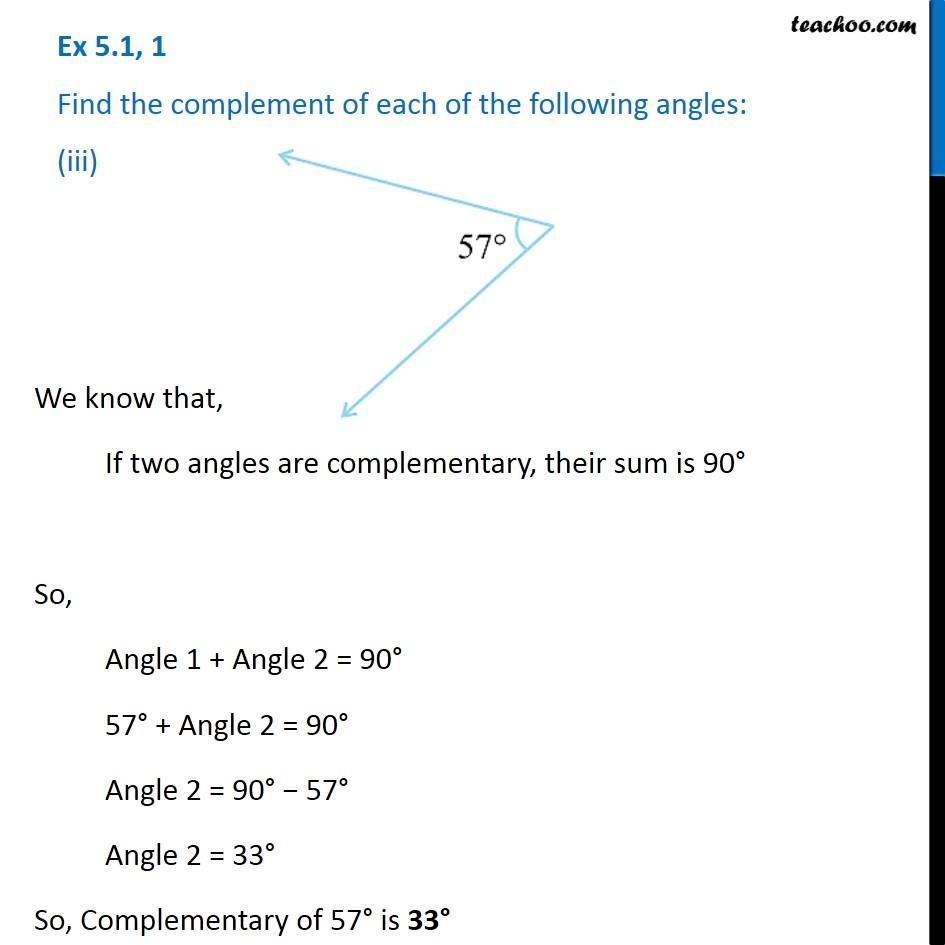Subscribe to our Youtube Channel - https://you.tube/teachoo

1. Chapter 5 Class 7 Lines and Angles
2. Serial order wise
3. Ex 5.1

Transcript

Ex 5.1, 1 Find the complement of each of the following angles: (i) We know that, If two angles are complementary, their sum is 90° So, Angle 1 + Angle 2 = 90° 20° + Angle 2 = 90° Angle 2 = 90° − 20° Angle 2 = 70° So, Complementary of 20° is 70° Ex 5.1, 1 Find the complement of each of the following angles: (ii) We know that, If two angles are complementary, their sum is 90° So, Angle 1 + Angle 2 = 90° 63° + Angle 2 = 90° Angle 2 = 90° − 63° Angle 2 = 27° So, Complementary of 63° is 27° Ex 5.1, 1 Find the complement of each of the following angles: (iii)We know that, If two angles are complementary, their sum is 90° So, Angle 1 + Angle 2 = 90° 57° + Angle 2 = 90° Angle 2 = 90° − 57° Angle 2 = 33° So, Complementary of 57° is 33°

Ex 5.1